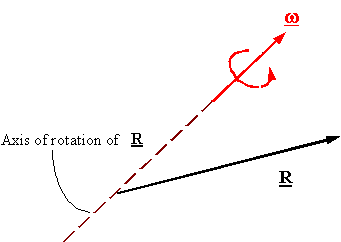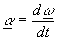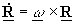Kinematics of Rigid bodies

Angular velocity: The angular velocity w of a vector R is itself a vector which has a magnitude equal to the rate of rotation, and is pointing along the axis of rotation of R, following the right-hand rule where the thumb is along the axis of rotation and the other fingers provide the sense in which R is rotating around the axis of rotation. The units of angular velocity are in radians over a suitable unit of time.Angular acceleration: The angular acceleration a is the rate of change of the angular velocity with respect to time.The derivative of a constant length vector (lemma): If the length of vector R is constant (|R| = constant), then one can calculate its derivative with respect to time using the formula